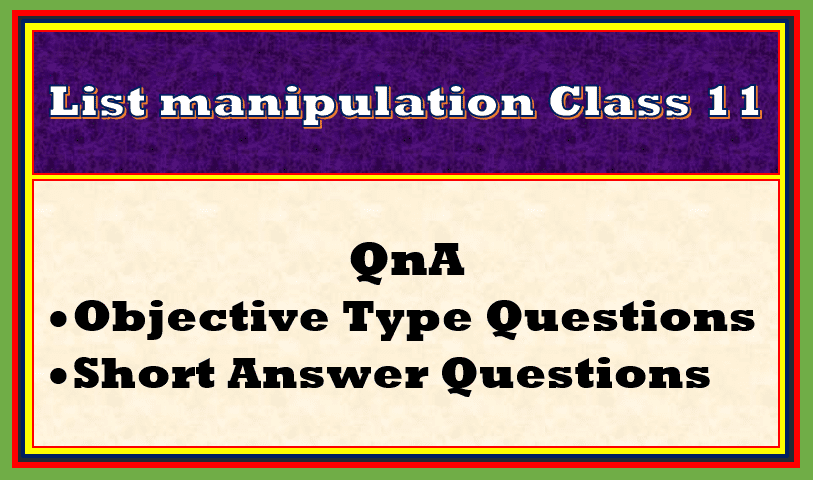In this article, we will discuss Important QnA List Manipulations class 11, do the practice and enjoy the learning!

Topics Covered

## Important QnA List Manipulations class 11

As usual we will start our important QnA session with objective type questions, so here we begin Important QnA List Manipulations class 11.

## Objective type questions

This section of Important QnA List Manipulations class 11 consists of objective type questions of one marks in the form of fill in the blanks, MCQs and True/False. Here we start!

1. Accessing list element through it’s index is known as traversing list. (True/False)
2. If the list index is starting with 0,1,2 and so on for the list, is known as __________ indexing.
3. To access list from reverse order is known as ___________ indexing.
4. You can traverse the list in for loop without range function. (True/False)
5. Slicing means displaying list elements from a start position to end position with specified step values. (True/False)
6. s1= l[0:4] will return
1. First four elements
2. First three elements
3. Elements between 0 and 4
4. All of these
7. s1=[1:-1] will return
1. First to the last element
2. Second to second last element
3. First to the second element
4. First index to index 2
8. What will be the output of l * 2, if l=[7,5]
1. [14,10]
2. [14,10,14,10]
3. [7,7,5,5]
4. [7,5,7,5]
9. If l1=[4,6] and l2=[3,5], what will the output of l1 + l2
1. [7,11]
2. [4,6,3,5]
3. [3,4,5,6]
4. [3,5,4,6]
10. s = l[:-1] will return,
1. a list in reverse order
2. a list in the same order but ignore the last element
3. a list in the reverse order and ignore the first element
4. all element with decrement value 1
11. Ms. Priya is working with the list. Now she wants to print the entire list in reverse order using slice. Which of the following statement will print the element in reverse order?
1. l[len(l-1),0]
2. l[-1:]
3. l[len(l1)-1,0]
4. l[::-1]
12. Suppose a list is having 7 elements. Then what will be the correct statement out of the following in case the slice is written in this manner: l[1:11]
1. Error
2. Ignore the first element and display the rest of all
3. Display the element starting from the second element to the length of elements and rest will be garbage values
4. No output, infinite loop
13. Ms. Suman wants to print only the last element of the list using slice. Which of the following statement is correct?
1. l
2. l[-1:]
3. l[:-1]
4. l[len(l)-1]
14. What will be the output of l[len(l)-2], if l = [12,23,34,45,56,67,78,89]:
1. 67
2. 23
3. 6
4. 78
15. Consider the same list given in the question no. 14 and what will be the output for the statement print(45 in l):
1. True
2. False
3. Error
4. 45
16. You cannot compare two lists if they are of different length. (True/False)
17. The ___________ keyword is used to make a true copy of the list.
18. What will be the output of l[-1][-1], if l=[‘Anand’,’Surat’,’Bhuj’,’Morbi’]:
1. Anand
2. Morbi
3. A
4. i
19. What will be the output of print(l) If l=[‘Virat’,[78,45,99]]:
1. V
2. i
3. 78
4. 45
20. What will be the output of print(l,l), consider the above list:
1. Virat 45
2. Virat 78
3. V 45
4. V 78 45

Now let us see the answer of the objective questions of QnA List Manipulations class 11.

1. True
2. positive
3. negative
4. True (By using in operator)
5. True
6. 1 First four elements
7. 2 Second to second last element
8. 4 [7,5,7,5]
9. 2 [4,6,3,5]
10. 2 a list in the same order but ignore the last element
1. 4 l[::-1]
2. 2 Ignore the first element and display the rest of all
3. 3 l[:-1]
4. 4 78
5. 1 True
6. False
7. copy
8. 4 i
9. 2 i
10. 1 Virat 45

Now let us discuss short answer questions for Important QnA List Manipulations class 11.

1. What do you mean by traversing a list? Explain with a suitable example.
2. Enlist the ways of traversing a list.
3. What do you mean by positive indexing and negative indexing? Explain with example.
4. How to traverse a list by using for loop? Explain with example.
5. How to traverse a list using membership operator in? Elaborate your answer with an example.
6. Explain the list slicing with example.
7. What is the difference between concatenation and replication operator?
8. What do you mean by making a true copy of a list?